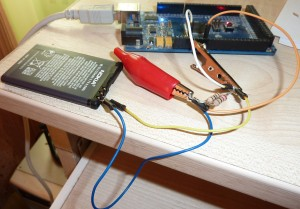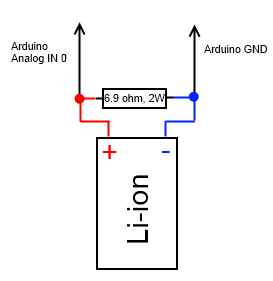# Very simple Arduino Lithium-ion battery capacity tester/discharge monitorThis is a very simple capacity tester. It consists of single resistor that discharges battery. Arduino measures the voltage drop across resistor. According to Ohm’s Law current = voltage/resistance. Every second value of current is divided by 3600 and summed up to get the capacity expressed in Ah (Amp per hour).I have used two parallel connected resistors that total resistance is 6.9 ohm. Make sure that they have proper power rating, if You don’t want them to convert to smoke. If voltage across 6.9 ohm resistor is  3.7 V, then current – 0.54 A, power ~ 2W.